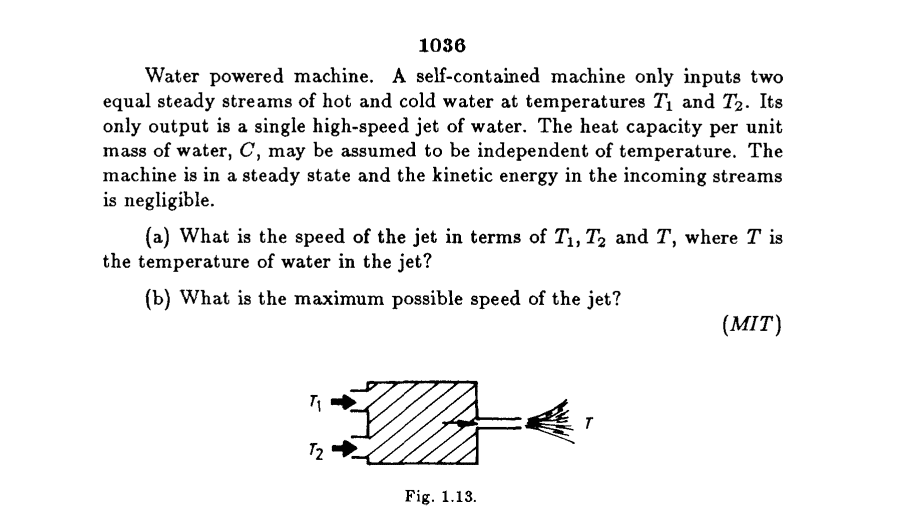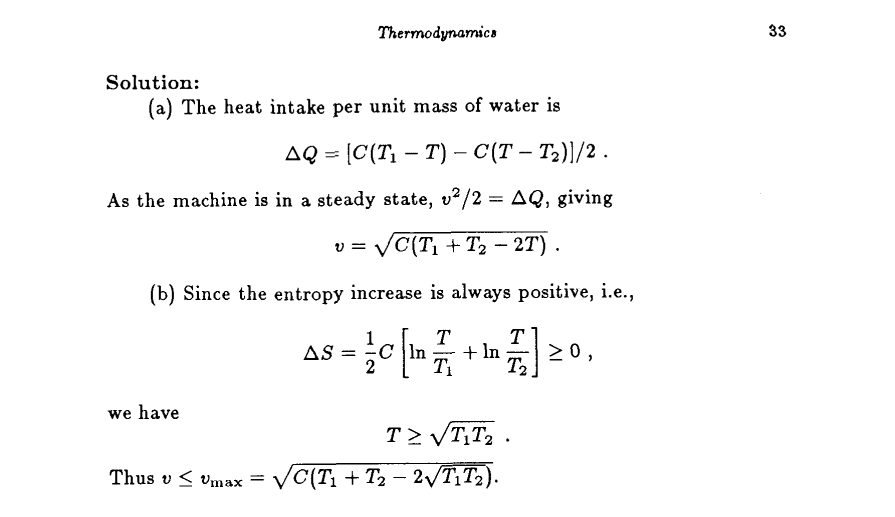# Heat transfer in thermodynamics

Homework Statement:
What is the speed of the jet in terms of T1,TZ and T
Relevant Equations:
delta Q = mC delta T
or
delta Q = c delta T , where c is specific heat capacityIn answering questions (a.) Why heat intake in this system is
## \Delta Q_{sys} = ( \Delta Q_{hot water} + \Delta Q_{cold water} ) / 2 ##
where.
##\Delta Q_{hot water} = c(T_1 - T_f) ##
##\Delta Q_{cold water} = c(T_2 - T_f) ##
I think T or T_f should be between T_1 and T_2
But why is the ##\Delta Q_{sys}## multiplied by a factor of 1/2?

Chestermiller
Mentor
Homework Statement:: What is the speed of the jet in terms of T1,TZ and T
Relevant Equations:: delta Q = mC delta T
or
delta Q = c delta T , where c is specific heat capacity

View attachment 278264View attachment 278265

In answering questions (a.) Why heat intake in this system is
## \Delta Q_{sys} = ( \Delta Q_{hot water} + \Delta Q_{cold water} ) / 2 ##
where.
##\Delta Q_{hot water} = c(T_1 - T_f) ##
##\Delta Q_{cold water} = c(T_2 - T_f) ##
I think T or T_f should be between T_1 and T_2
But why is the ##\Delta Q_{sys}## multiplied by a factor of 1/2?
This question and its solution makes no sense to me. Where did the equation between ##\Delta Q## (whatever that is) and v come from?

This question and its solution makes no sense to me. Where did the equation between ΔQ (whatever that is) and v come from?

I think because it is Water powered machine and Its
only output is a single high-speed jet of water.
so kinetic energy may be equal to heat energy.
It could be inferred that 1/2mv^2 = delta Q
So "v" is the speed of this machine.

Chestermiller
Mentor
So you are saying that, from the open system (control volume) version of the 1st law of thermodynamics, the decrease in enthalpy per unit mass of the liquid streams is equal to the increase in kinetic energy per unit mass of the liquid streams.. And, from the open system version of the 2nd law of thermodynamics, the change in entropy of the liquid streams is greater than zero.

BTW, Q is not heat in these equations. It is enthalpy H, and the symbol H should properly be used.

Also, that final equation has an exact square under the square root sign.

Steve4Physics
Homework Helper
Gold Member
But why is the ##\Delta Q_{sys}## multiplied by a factor of 1/2?
First, I'll note that I'm not entirely clear how such a machine would work. I guess if the volume expansion coefficient of water were not constant over the temperature range, a pressure-change could result from the mixing. This would result in work being done, accelerating the water through the jet. Something like that, anyway.

I think the text book solution is correct but unclear. The logic gets ‘muddied’ by the fact that ##c(T_1-T)## and ##c(T-T_2)## are both energy per kg.

If ##c(T_1-T) > c(T-T_2)## then ##c(T_1-T) – c(T-T_2)## is the energy available to do work on ***two (not one)*** kg of water (1kg of hot water mixed with 1kg of cold water). So half this amount is the energy available to do work on 1kg of water. That's where the factor of ½ comes from.

I’d present the the solution slightly differently:

Say m kg of hot water and m kg of cold water are mixed, Then 2m kg of water comes out of the jet. We can write directly:
##mc(T_1-T) - mc(T-T_2) = ½(2m)v^2##
which gives the required speed without worrying about the factor ½.

•Another
Chestermiller
Mentor
First, I'll note that I'm not entirely clear how such a machine would work. I guess if the volume expansion coefficient of water were not constant over the temperature range, a pressure-change could result from the mixing. This would result in work being done, accelerating the water through the jet. Something like that, anyway.
This is not a machine, and there is no volume change or increase. It is just a simple mixing device in which the outlet cross sectional area is much less than the cross sectional areas of the inlets. That's how you get a jet.

•Another
Chestermiller
Mentor
The way I would formulate the open system version of the 1st law of thermo for this mixer would be (per unit mass of flow through the mixer), $$\Delta \left(h+\frac{v^2}{2}\right)=0$$where $$\Delta h=\frac{C(T-T_1)+C(T-T_2)}{2}$$ and where the inlet velocity is essentially zero. The open system version of the 2nd law, per unit mass of flow through the mixer would be: $$\Delta s=\frac{C\ln{(T/T_1)}+C\ln{(T/T_2)}}{2}=\sigma$$where ##\sigma## is the entropy generated per unit mass of fluid passing through the adiabatic mixer. So we would have $$T=\sqrt{T_1T_2}\exp{\left(\frac{2\sigma}{C}\right)}$$So we would have $$v^2=C\left(T_1+T_2-2\sqrt{T_1T_2}\exp{\left(\frac{2\sigma}{C}\right)}\right)$$The upper bound to v would be if ##\sigma=0##, in which case $$v=\sqrt{C}\ |\sqrt{T_1}-\sqrt{T_2}\ |$$

Steve4Physics
Homework Helper
Gold Member
This is not a machine, and there is no volume change or increase. It is just a simple mixing device in which the outlet cross sectional area is much less than the cross sectional areas of the inlets. That's how you get a jet.
I used the word ‘machine’ simply for consistency with the textbook, which calls the system a ‘machine’ (twice!).

Also, I didn’t mention any ‘volume’ increase. I was simply trying to conceive of a physical mechanism that could make v a function of the jet's temperature.

The question describes a very unrealistic/impractical system purely (I guess) as a teaching/learning exercise.

I will note that my interpretation explains the textbook solution and gives the required answer:
##½(2m)v^2 = mc(T_1-T) – mc(T-T_2)##
##v = \sqrt{c(T_1-T) – c(T-T_2)} = \sqrt{c(T_1 + T_2-2T)}##

I understand your explanation of how a jet is produced – but it does not explain why the jet’s speed is a function of the jet's temperature T (which is surely the essence of the question).

Chestermiller
Mentor
I used the word ‘machine’ simply for consistency with the textbook, which calls the system a ‘machine’ (twice!).

Also, I didn’t mention any ‘volume’ increase. I was simply trying to conceive of a physical mechanism that could make v a function of the jet's temperature.

The question describes a very unrealistic/impractical system purely (I guess) as a teaching/learning exercise.

I will note that my interpretation explains the textbook solution and gives the required answer:
½½(2m)v2=mc(T1−T)–mc(T−T2)
v=c(T1−T)–c(T−T2)=c(T1+T2−2T)

I understand your explanation of how a jet is produced – but it does not explain why the jet’s speed is a function of the jet's temperature T (which is surely the essence of the question).
I totally agree. I seems obvious to me that the volume flow rate has to come in.

Worse yet, the final result of this analysis says that, if the two inlet temperatures are equal, the upper bound to the jet velocity is zero, which is silly.

Last edited:
Chestermiller
Mentor
If I were analyzing this system, I would judge that the only realistic way that the exit temperature is different from the arithmetic average of the two entrance temperatures is if there is a significant pressure drop accompanied by viscous heating. In this case, neglecting thermal expansion of the liquid (i.e., treating the liquid as incompressible), we would have $$\Delta h=C_p\Delta T+V\Delta P$$ where V is the specific volume of the liquid. So, neglecting the kinetic energy of the jet, we would have $$\frac{C}{2}(2T-T_1-T_2)=V(-\Delta P)-\frac{v^2}{2}$$or $$T=\frac{(T_1+T_2)}{2}+\frac{[V(-\Delta P)-\frac{v^2}{2}]}{C}$$
The Bernoulli Equation tells us that, for an inviscid fluid, ##V(-\Delta P)-\frac{v^2}{2}=0##. This means that for a viscous dissipative fluid, this quantity is going to be positive.

Applying the open system version of the 2nd law of thermodynamics to this system now tells us that $$\Delta s=C\ln{\left[\frac{(T_1+T_2)}{2\sqrt{T_1T_2}}+\frac{[V(-\Delta P)-\frac{v^2}{2}]}{C\sqrt{T_1T_2}}\right]}$$
Both terms in brackets are going to be positive, giving rise to a. positive change in entropy, irrespective of the jet velocity. The first term represents the contribution of thermally mixing the two input streams and the second term represents the contribution of viscous dissipation.

This analysis indicates that the term that was missing from the OP's posted MIT bogus analysis was the ##V\Delta P## term, which is critical to what is happening in the jet device, and which now makes the results have some sense.

Last edited: